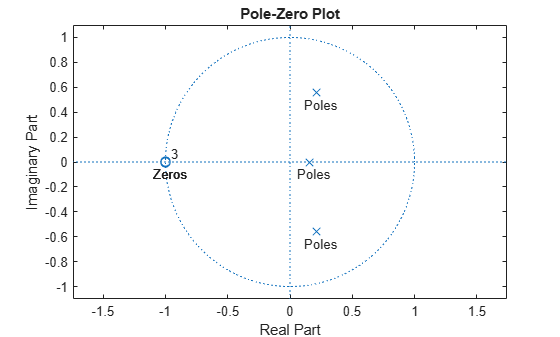Documentation

# tf2zpk

Convert transfer function filter parameters to zero-pole-gain form

## Syntax

``[z,p,k] = tf2zpk(b,a)``

## Description

example

````[z,p,k] = tf2zpk(b,a)` finds the matrix of zeros `z`, the vector of poles `p`, and the associated vector of gains `k` from the transfer function parameters `b` and `a`. The function converts a polynomial transfer-function representation $H\left(z\right)=\frac{B\left(z\right)}{A\left(z\right)}=\frac{{b}_{1}+{b}_{2}{z}^{-1}\cdots +{b}_{n-1}{z}^{-n}+{b}_{n}{z}^{-n-1}}{{a}_{1}+{a}_{2}{z}^{-1}\cdots +{a}_{m-1}{z}^{-m}+{a}_{m}{z}^{-m-1}}$of a single-input/multi-output (SIMO) discrete-time system to a factored transfer function form $H\left(z\right)=\frac{Z\left(z\right)}{P\left(z\right)}=k\frac{\left(z-{z}_{1}\right)\left(z-{z}_{2}\right)\cdots \left(z-{z}_{m}\right)}{\left(z-{p}_{1}\right)\left(z-{p}_{2}\right)\cdots \left(z-{p}_{n}\right)}.$ NoteUse `tf2zpk` when working with transfer functions expressed in inverse powers (1 + z–1 + z–2). A similar function, `tf2zp`, is more useful for working with positive powers (s2 + s + 1), such as in continuous-time transfer functions. ```

## Examples

collapse all

Design a 3rd-order Butterworth filter with normalized cutoff frequency $0.4\pi$ rad/sample. Find the poles, zeros, and gain of the filter.

```[b,a] = butter(3,.4); [z,p,k] = tf2zpk(b,a)```
```z = 3×1 complex -1.0000 + 0.0000i -1.0000 - 0.0000i -1.0000 + 0.0000i ```
```p = 3×1 complex 0.2094 + 0.5582i 0.2094 - 0.5582i 0.1584 + 0.0000i ```
```k = 0.0985 ```

Plot the poles and zeros to verify that they are where expected.

```fvtool(b,a,'polezero') text(real(z)-0.1,imag(z)-0.1,'\bfZeros','color',[0 0.4 0]) text(real(p)-0.1,imag(p)-0.1,'\bfPoles','color',[0.6 0 0])```## Input Arguments

collapse all

Transfer function numerator coefficients, specified as a vector or matrix. If `b` is a matrix, then each row of `b` corresponds to an output of the system. `b` contains the coefficients in descending powers of z. The number of columns of `b` must be equal to the length of `a`. If the numbers differ, make them equal by padding zeros. You can use the function `eqtflength` to accomplish this.

Data Types: `single` | `double`

Transfer function denominator coefficients, specified as a vector. `a` contains the coefficients in descending powers of z.

Data Types: `single` | `double`

## Output Arguments

collapse all

Zeros of the system, returned as a matrix. `z` contains the numerator zeros in its columns. `z` has as many columns as there are outputs.

Poles of the system, returned as a column vector. `p` contains the pole locations of the denominator coefficients of the transfer function

Gains of the system, returned as a column vector. `k` contains the gains for each numerator transfer function.